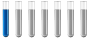## need some help with these questions

Chemistry and homework help forum.

Organic Chemistry, Analytical Chemistry, Biochemistry, Physical Chemistry, Computational Chemistry, Theoretical Chemistry, High School Chemistry, Colledge Chemistry and University Chemistry Forum.

Share your chemistry ideas, discuss chemical problems, ask for help with scientific chemistry questions, inspire others by your chemistry vision!

Please feel free to start a scientific chemistry discussion here!

Discuss chemistry homework problems with experts!

Ask for help with chemical questions and help others with your chemistry knowledge!

Moderators: Xen, expert, ChenBeier

eng
NewbiePosts: 1
Joined: Thu Jun 09, 2022 10:56 am

### need some help with these questions

1.How many grams of benzoic acid (C6H5COOH) must be dissolved in 250 mL of water to have a solution with pH of 3.02. Ka=3x10^-5
your experiment, you need 2.1 L of a solution with a pH of 3.50. How many mL of 2 mM H2SO4 solution you need to use to prepare the desired solution?
3. Calculate the pH, [H3O+] and [S04] of 0.55 M H2SO4 solution? (Kaz: 1.1x10-2)
ChenBeier
Distinguished MemberPosts: 1172
Joined: Wed Sep 27, 2017 7:25 am
Location: Berlin, Germany

### Re: need some help with these questions

1. Formula of weak acids pH = ½( pKa - log [c HA ] 0 )
2. Calculate cH+ of 2.1 l pH 3.5, this amount has to be delivered from 2 mM H2SO4.
3.We consider the example of sulfuric acid, cS = 0.01 mol/l.

1st stage

H2SO4 => HSO4- + H+

practically 100% - strong acid

2nd stage

HSO4- => SO42- + H+

Equilibrium, pKa = 1.92 - weak acid

The calculation of the equilibria (all particle types) leads to the following solution:
pH = 1.838; c(H2SO4) = 7.9*10-8 (0%); c(HSO4-) = 5.5*10-3 (55%); c(SO42-) = 4.5*10-3 (45%).
>  A calculation would be wrong: cS = 0.01; pH = - log( cS ) = 2. (Only the first stage considered)
>  One calculation is also wrong: Sulfuric acid is bivalent, so 1 H2SO4 produces 2 H+;
for cS = 0.01 we have c( H+ ) = 2 * cS = 0.02; pH = - log( 0.02 ) = 1.699.
>  A correct solution with "chemical considerations" would be:
The 1st stage of dissociation is complete; 0.01 mol/l H2SO4 therefore produces 0.01 mol/l H+ and 0.01 mol/l HSO4-.
For the 2nd stage we have an equilibrium: KS = 10-1.92 = 0.01202 = c( H+ ) * c( SO42- ) / c( HSO4- ).
If a portion x of HSO4- dissociates: x SO42- are formed, c( HSO4- ) = 0.01 decreases by x
and c( H+ ) = 0.01 increases by x: KS = [ 0.01 + x ] * x / [ 0.01 - x ]
The (positive) solution of the quadratic equation x2 + ( 0.01 + KS ) * x - KS * 0.01 = 0 is: x = 0.004528.
From this follows the result: c( H+ ) = 0.014528; pH = 1.838; c(HSO4-) = 5.5*10-3; c( SO42- ) = 4.5*10-3.